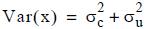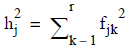# 5.4.5 - Principal Axis Factors - Teradata Warehouse Miner

## Teradata Warehouse Miner User Guide - Volume 3Analytic Functions

Product
Release Number
5.4.5
Published
February 2018
Language
English (United States)
Last Update
2018-05-04
dita:mapPath
yuy1504291362546.ditamap
dita:ditavalPath
ft:empty

In order to talk about principal axis factors (PAF) the term communality must first be introduced. In the common factor model, each original variable x is thought of to be a combination of common factors and a unique factor. The variance of x can then also be thought of as being composed of a common portion and a unique portion, that is. It is the common portion of the variance of x that is called the communality of x, that is the variance that the variable has in common through the common factors with all the other variables.

In the algorithm for principal axis factors described below it is of interest to both make an initial estimate of the communality of each variable, and to calculate the actual communality for the variables in a factor model with uncorrelated factors. One method of making an initial estimate of the communality of each variable is to take the largest correlation of that variable with respect to the other variables. The preferred method however is to calculate its squared multiple correlation coefficient with respect to all of the other variables taken as a whole. This is the technique used by Teradata Warehouse Miner. The multiple correlation coefficient is a measure of the overall linear association of one variable with several other variables, that is, the correlation between a variable and the best-fitting linear combination of the other variables. The square of this value has the useful property of being a lower bound for the communality. Once a factor model is built, the actual communality of a variable is simply the sum of the squares of the factor loadings, i.e.,With the idea of communality thus in place it is straightforward to describe the principal axis factors algorithm. Begin by estimating the communality of each variable and replacing this value in the appropriate position in the diagonal of the correlation or covariance matrix being factored. Then a principal components solution is found in the usual manner, as described earlier. As before, the user has the option of specifying either a fixed number of desired factors or a minimum eigenvalue by which to reduce the number of factors in the solution. Finally, the new communalities are calculated as the sum of the squared factor loadings, and these values are substituted into the correlation or covariance matrix. This process is repeated until the communalities change by only a small amount.

Through its use of communality estimates, the principal axis factor method attempts to find independent common factors that account for the covariance or correlation between the original variables in the model, while ignoring the effect of unique factors. It is then possible to use the factor loadings matrix to reproduce the correlation or covariance matrix and compare this to the original as a way of assessing the effectiveness of the model. The reproduced correlation or covariance matrix is simply the factor loadings matrix times its transpose (i.e., CCT). The user may optionally request that the signs of the factor loadings be inverted if there are more minus signs than positive ones. This is purely cosmetic and does not affect the solution in a substantive way. However, if signs are reversed, this must be kept in mind when attempting to interpret or assign meaning to the factors.